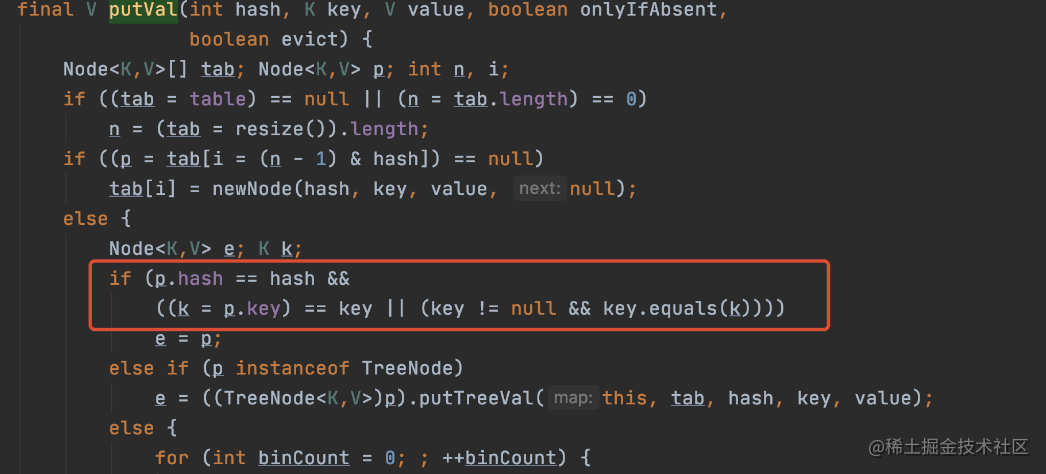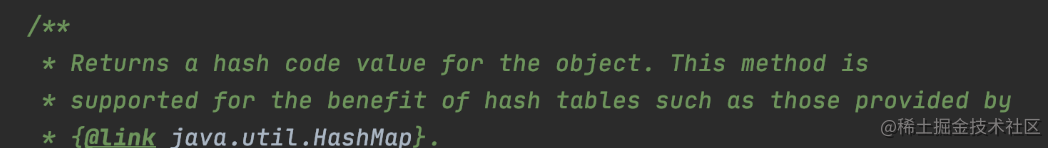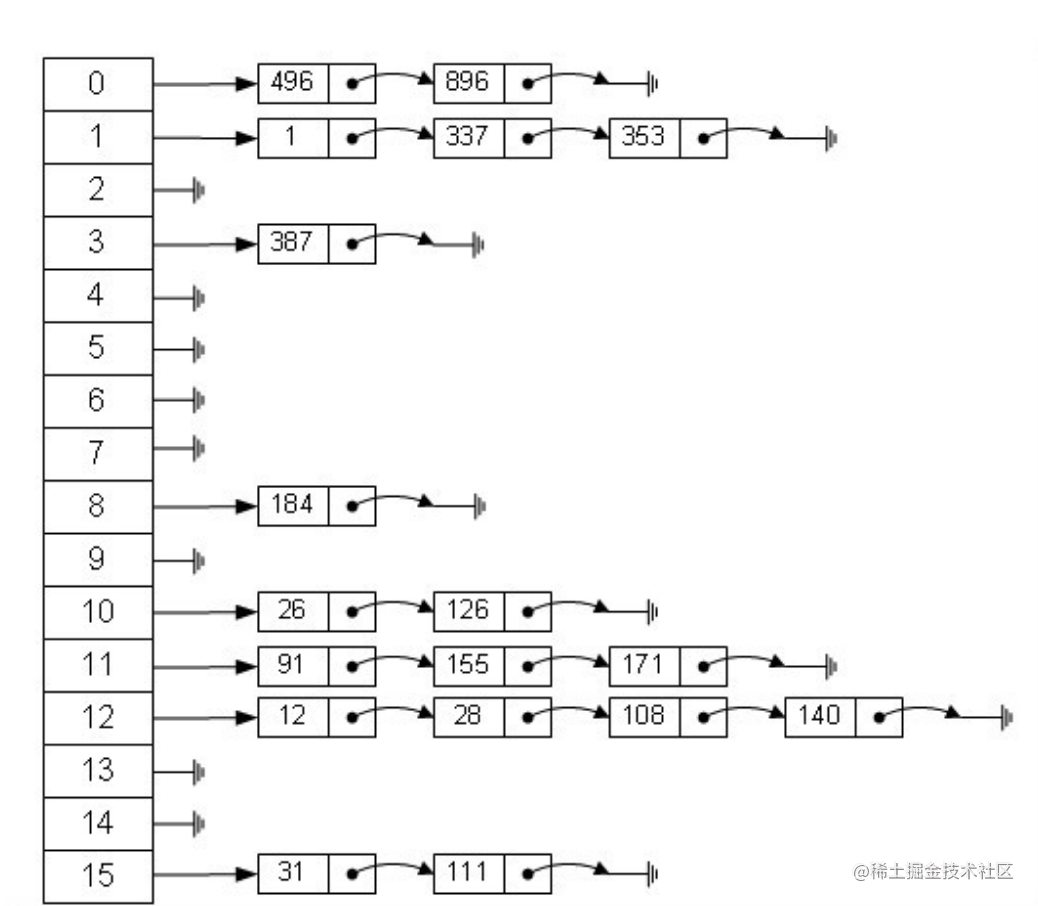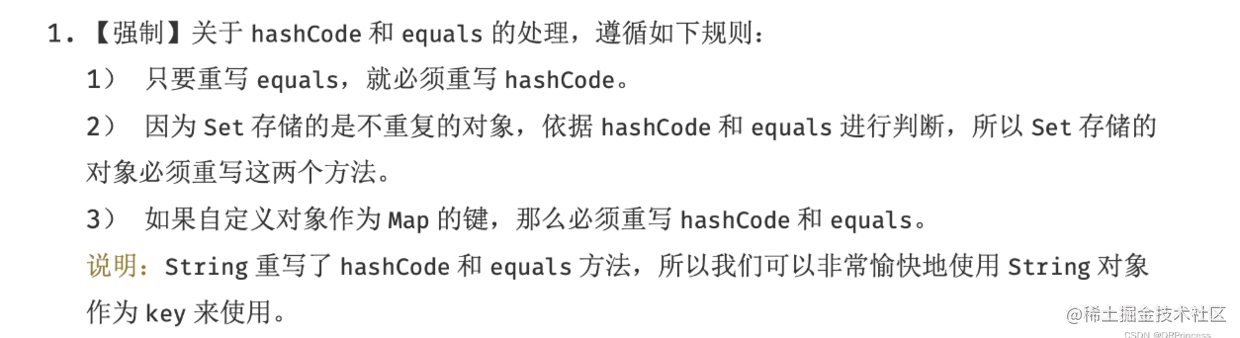# 面试全经过&题目

```java public class Test { class Item{

``````    private int code = 1;

public Item(int code){
this.code =  code;
}

override public int hashCode() {
return code;
}
}

public static void main(String[] args) {
Set<Item> set = new HashSet<>();

System.out.println(set.size());

}
``````

}

```

• 面试官： 这有一道代码题，你看下，你觉得最终会输出什么呢？
• 我：2
• 面试官：为什么？谈谈原因？
• 我：因为重写了hash,HashSet 内部会认为传递同样的code是同一个对象，所以第一个`new Item(0)`和第二个`new Item(0)` 会被认为是同一个对象，然后后者会覆盖前者，所以是2

• 面试官：实际上这段代码是不能运行的，现在需要你修改正确，并输出结果

• 我：编写代码中....(Item 改成了 static,override 关键字修改为 @Override注释)
• 我：改完了，运行一看，发现是 3，你说尴尬不尴尬.....
• 面试官：你觉得为啥和你之前设想的不一样
• 我：应该是还需要实现equals方法
• 面试官：为什么呢？
• 我：...

# 问题解惑

2. hashCode() 和 equals() 的理解

``````//固定的value值
private static final Object PRESENT = new Object();
``````

``````//添加方法，实际上，将数据作为key存储到  HashMap 中
return map.put(e, PRESENT)==null;
}
``````

} ```看下分支条件

```if (p.hash == hash && ((k = p.key) == key || (key != null && key.equals(k))))```

• p.hash == hash

```//put 的时候进行hash() public V put(K key, V value) { return putVal(hash(key), key, value, false, true); } //hash算法的实现 static final int hash(Object key) { int h; return (key == null) ? 0 : (h = key.hashCode()) ^ (h >>> 16); }```

• (k = p.key) == key || (key != null && key.equals(k))

## hashCode() 和 equals() 的理解

### 首先它们是什么？

```

``````public boolean equals(Object obj) {
return (this == obj);
}

@HotSpotIntrinsicCandidate
public native int hashCode();
``````

```

equals() ：内部使用了 “==”。判断是否是同个对象，如果是值类型，判断内容是否一致，如果是引用类型，则是引用地址是否相等。 hashCode()：一个本地方法，主要看native 中的实现。

1. 随机数
2. 内存地址做移位再和一个随机数做异或
3. 固定值1
4. 自增序列的当前值
5. 内存地址
6. 当前线程有关的一个随机数+三个确定值，运用xorshift随机数算法得到的一个随机数。

### 然后看为什么？两个方法的设计目的• equals() 的默认实现是用的内存地址，但是也有很多类改写了，例如 String 就修改了。
• equals() 允许重写后，逻辑可能会变得复杂，所以就性能上来说，大概率没有 hashCode() 的性能高。

```if (p.hash == hash && ((k = p.key) == key || (key != null && key.equals(k))))```

### 然后怎么用？重写 equals() 和 hashCode() 的注意事项

``` // String.java public boolean equals(Object anObject) { //内存地址相等，肯定是同个对象 if (this == anObject) { return true; } //否则逐个判断字符是否一致 if (anObject instanceof String) { String aString = (String)anObject; if (!COMPACT_STRINGS || this.coder == aString.coder) { return StringLatin1.equals(value, aString.value); } } return false; }

``````public int hashCode() {
int h = hash;
if (h == 0 && !hashIsZero) {
h = isLatin1() ? StringLatin1.hashCode(value)
: StringUTF16.hashCode(value);
if (h == 0) {
hashIsZero = true;
} else {
hash = h;
}
}
return h;
}
``````

//StringLatin1.java

``````//哈希算法的真正实现
public static int hashCode(byte[] value) {
int h = 0;
for (byte v : value) {
h = 31 * h + (v & 0xff);
}
return h;
}

//逐个判断字符是否一致
public static boolean equals(byte[] value, byte[] other) {
if (value.length == other.length) {
for (int i = 0; i < value.length; i++) {
if (value[i] != other[i]) {
return false;
}
}
return true;
}
return false;
}
``````

```

equals()重写的时候需要满足以下规则：

• 内存地址相同，一定返回true (使用“==”操作符检查)
• 类型不一致，一定返回false(使用instanceof 操作符检查)
• 类型转换后，判断类中每个关键属性，符合预设，返回 true , 否则 false

hashCode()重写的时候需要满足以下规则：

• 保证同一个对象，多次调用 Hashcode 是一致的。
• equals()相等，hashCode()肯定相等# 总结

1. 不受重写 hashCode() 的干扰，给出正确的列表长度答案
2. 说出对 hashCode() 和 equals() 的理解

# 参考资料

Effective Java 中文版

Java Object.hashCode()返回的是对象内存地址？